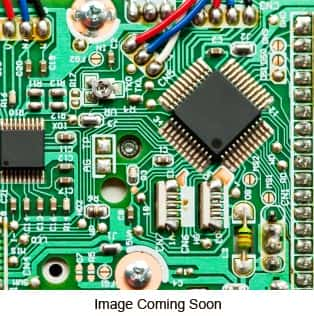# CA3028A CA3028 A Bipolar Differential Amplifier ICManufacturer: RCA
Part Number: CA3028A

Price: \$4.25
Sorry, we are currently sold out of
'CA3028A CA3028 A Bipolar Differential Amplifier IC'.
Code: T383PD
Quantity:

Bipolar Differential/Cascode Amplifiers IC's
8 Pin Metal Can Package
Package Number: T8.C

Electrical Characteristics (typ. @ TA=25�C unless otherwise noted):
DC Characteristics:
Input Bias Current: 16.6�A
(VCC = 6V, VEE = -6V)
Input Bias Current: 36�A
(VCC = 12V, VEE = -12V)
Quiescent Operating Current: 1.25mA
(VCC = 6V, VEE = -6V)
AGC Bias Current(Into Constant Current Source Terminal 7): 1.28mA
(VCC = 12V, VAGC = 9V)
Input Current(Terminal 7): .85mA
(VCC = 6V, VEE = -6V)
Power Dissipation: 36mW
(VCC = 6V, VEE = -6V)

Dynamic Characteristics:
Power Gain: 20dB Cascode ; 17dB Diff Amp.
(f = 100MHz, VCC = 9V)
Noise Figure: 7.2dB Cascode ; 6.7dB Diff Amp.
(f = 100MHz, VCC = 9V)